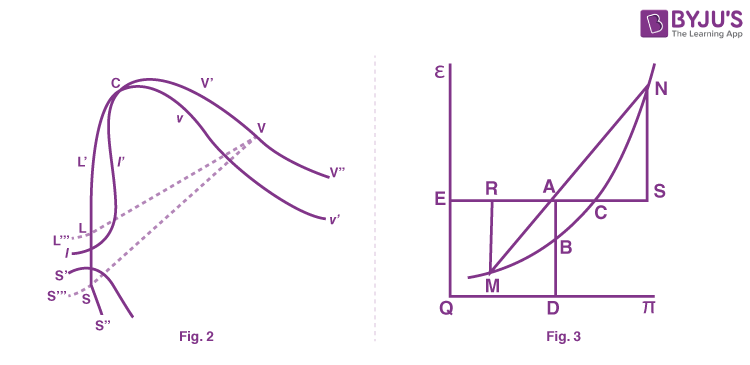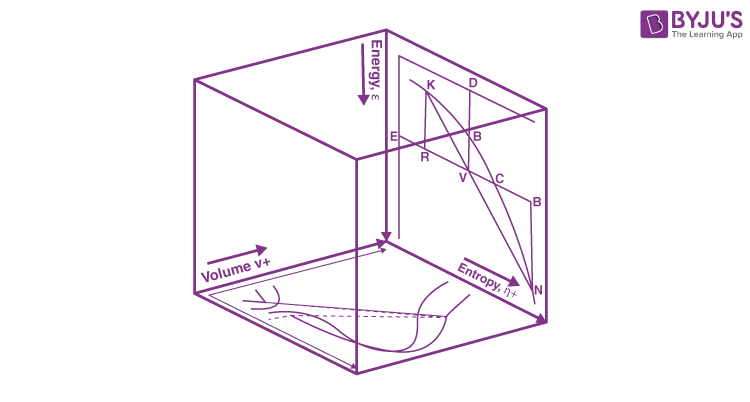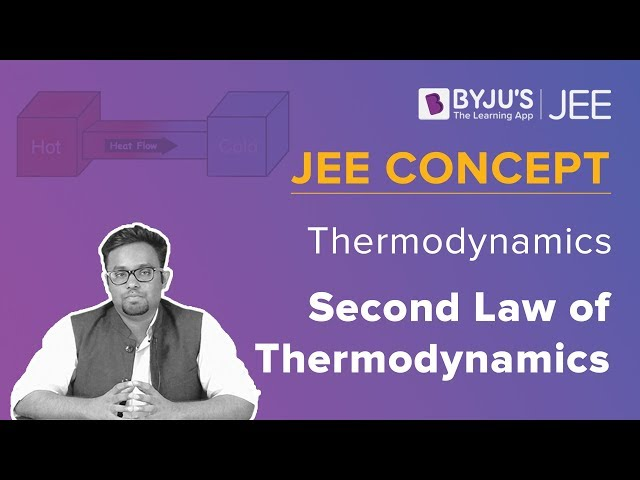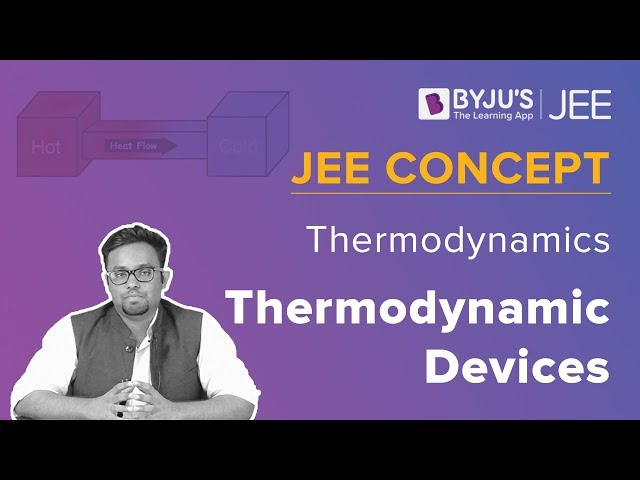Win up to 100% scholarship on Aakash BYJU'S JEE/NEET courses with ABNAT Win up to 100% scholarship on Aakash BYJU'S JEE/NEET courses with ABNAT

# Gibbs Free Energy

Gibbs free energy, also known as the Gibbs function, Gibbs energy, or free enthalpy, is a quantity that is used to measure the maximum amount of work done in a thermodynamic system when the temperature and pressure are kept constant. Gibbs free energy is denoted by the symbol ‘G’. Its value is usually expressed in Joules or Kilojoules. Gibbs free energy can be defined as the maximum amount of work that can be extracted from a closed system.

This property was determined by American scientist Josiah Willard Gibbs in the year 1876 when he was conducting experiments to predict the behaviour of systems when combined together or whether a process could occur simultaneously and spontaneously. Gibbs free energy was also previously known as “available energy.” It can be visualised as the amount of useful energy present in a thermodynamic system that can be utilised to perform some work.

## Gibbs Free Energy Equation

Gibbs free energy is equal to the enthalpy of the system minus the product of the temperature and entropy. The equation is given as:

G = H – TS

Where,

G = Gibbs free energy

H = enthalpy

T = temperature

S = entropy

OR

or more completely as:

G = U + PV – TS

Where,

• U = internal energy (SI unit: joule)
• P = pressure (SI unit: pascal)
• V = volume (SI unit: m3)
• T = temperature (SI unit: kelvin)
• S = entropy (SI unit: joule/kelvin)

### Variations of the Equation

Gibbs free energy is a state function; hence it doesn’t depend on the path. So, change in Gibbs free energy is equal to the change in enthalpy minus the product of temperature and entropy change of the system.

ΔG = ΔH – Δ(TS)

If the reaction is carried out under constant temperature {ΔT=O}

ΔG = ΔH – TΔS

This equation is called the Gibbs-Helmholtz equation.

ΔG > 0; the reaction is non-spontaneous and endergonic

ΔG < 0; the reaction is spontaneous and exergonic

ΔG = 0; the reaction is at equilibrium

Note:

1. According to the second law of thermodynamics, the entropy of the universe always increases in a spontaneous process.
2. ΔG determines the direction and extent of chemical change.
3. ∆G is meaningful only for reactions in which the temperature and pressure remain constant. The system is usually open to the atmosphere (constant pressure), and we begin and end the process at room temperature (after any heat that we have added or which is liberated by the reaction has dissipated).
4. ∆G serves as the single master variable that determines whether a given chemical change is thermodynamically possible. Thus, if the free energy of the reactants is greater than that of the products, the entropy of the world will increase when the reaction takes place as written, and so the reaction will tend to take place spontaneously. ΔS universe = ΔS system + ΔS surroundings
5. If ΔG is negative, the process will occur spontaneously and is referred to as exergonic.
6. Therefore, spontaneity is dependent on the temperature of the system.
 Point to Remember Free energy change criteria for predicting spontaneity is better than entropy change criteria because the former requires free energy change of system only, whereas the latter requires entropy change of system and surroundings.

## Standard Energy Change of Formation

We can say that the standard Gibbs free energy of the formation of a compound is basically the change of Gibbs free energy that is followed by the formation of 1 mole of that substance from its component element available at their standard states or the most stable form of the element which is at 25 °C and 100 kPa. Its symbol is ΔfG˚.

All elements in their standard states (diatomic oxygen gas, graphite, etc.) have standard Gibbs free energy change of formation equal to zero, as there is no change involved.

ΔfG = ΔfG˚ + RT ln Qf,

where Qf is the reaction quotient.

At equilibrium, ΔfG = 0, and Qf = K, so the equation becomes

ΔfG˚ = −RT ln K,

Where K is the equilibrium constant.

## Graphical interpretation by Gibbs

Interestingly, Gibbs free energy was originally defined graphically. Willard Gibbs, in 1873, published his first thermodynamics paper titled “Graphical Methods in the Thermodynamics of Fluids.” In this paper, Gibbs used the two coordinates of entropy and volume to represent the state of the body. In addition to this, Gibbs, in his second follow-up paper, which was published later that year and titled “A Method of Geometrical Representation of the Thermodynamic Properties of Substances by Means of Surfaces.” added the third coordinate of the energy of the body, defined on three figures.In the following year, in 1874, a Physicist from Scotland named James Clerk Maxwell used Gibbs’ figures as a reference to make a 3D energy-entropy-volume thermodynamic surface of a fictitious water-like substance. Maxwell’s 3D surface figure is as follows:## Second Law of Thermodynamics

Based on concepts of entropy and spontaneity, the second law of thermodynamics is defined on the following basis:

1. All spontaneous processes are thermodynamically irreversible.
2. It is impossible to convert heat completely into work without wastage.
3. The entropy of the universe is continuously increasing.
4. The total entropy change, i.e., entropy change of the system + entropy change of surroundings, is positive.
 ∆H<0 ∆H>0 ∆S>0 Spontaneous at all temperatures (∆G<0) Spontaneous only at high temperature (when T∆S is high) ∆S<0 Spontaneous at low temperature (when T∆S is small) Not spontaneous at any temperature (∆G>0)

● Spontaneous – It is considered to be natural because it is a reaction that occurs by itself without any external action towards it.

● Non-spontaneous – It needs constant external energy applied to it in order for the process to continue, and once you stop the external action, the process will cease.

Standard-state free energy of reaction (∆G°)

The free energy of reaction at standard state conditions:

∆G°=∆H°-T∆S°

Standard-state Conditions

1. The partial pressure of any gases involved in the reaction is 0.1 MPa.
2. The concentrations of all aqueous solutions are 1 M.
3. Measurements are also generally taken at a temperature of 25C (298 K).
4. Pure liquids: The liquid under a total (hydrostatic) pressure of 1 atm.
5. Solids: The pure solid under 1 atm pressure.

The change in the free energy of the system that occurs during a reaction measures the balance between the two driving forces that determine whether a reaction is spontaneous. As we have seen, the enthalpy and entropy terms have different sign conventions.

 Favourable Unfavourable ∆H°<0 ∆H°>0 ∆S°>0 ∆S°<0

## Calculating the Change in Gibbs Free Energy

Even though ∆G is temperature-dependent, we assume to take ∆H and ∆S are independent of temperature when there is no phase change in the reaction. So, if we know ∆H and ∆S, we can find out the ∆G at any temperature.

Methods

1. Estimating ∆H reaction using bond enthalpies
2. Calculating ∆H using standard heats of formation ∆fH°
3. Calculating ∆H and ∆S using standard values

Tips:

● Unit of ∆G is equal to ∆H

● Unit of ∆H is (KJ) /(mole reaction)

● Unit of ∆S is (KJ) /(mole reaction)K

Examples

The rusting of iron is an example of a spontaneous reaction that occurs slowly, little by little, over time. The melting of ice is also an example of this.

## Relationship between Free Energy and Equilibrium Constant

The free energy change of the reaction in any state, ΔG (when equilibrium has not been attained), is related to the standard free energy change of the reaction, ΔG° (which is equal to the difference in the free energies of formation of the products and reactants, both in their standard states) according to the equation.

ΔG = ΔG° + RT InQ

Where Q is the reaction quotient.

At equilibrium,

∆G = 0, and Q become equal to the equilibrium constant. Hence, the equation becomes,

ΔG° = –RT In K(eq)

ΔG° = –2.303 RT log K(eq)

● R = 8.314 J mol-1 K-1 or 0.008314 kJ mol-1 K-1.

● T is the temperature on the Kelvin scale

In a reversible reaction, the free energy of the reaction mixture is lower than the free energy of reactants as well as products. Hence, free energy decreases whether we start from reactants or products, i.e., ∆G is -ve in backward as well as forward reactions.

## Relationship between Gibbs Free Energy and EMF of a Cell

In the case of galvanic cells, Gibbs energy change ΔG is related to the electrical work done by the cell.

ΔG = -nFE(cell)

Where, n = No. of moles of electrons involved

E = emf of the cell

F = 1 Faraday = 96500 coulombs

If reactants and products are in their standard states,

ΔG°= –nFE°cell

∆G°and equilibrium

 ∆G° Reaction Keq + Non-spontaneous <<1 – Spontaneous >>1 0 At equilibrium 0

## Gibbs Free Energy Problems

(1) Predict whether the following reaction is still spontaneous at 500 °C: N2(g) + 3 H2(g) ⇔2 NH3(g). Assume that H = 92.22 kJ mol-1 and S = -198.75 J K-1 mol-1

Solution:

Before we can decide whether the reaction is still spontaneous, we need to calculate the temperature of the Kelvin scale:

T = 500° C + 273 = 773 K

We then multiply the entropy term by this temperature and subtract this quantity from the enthalpy term:

∆G° = ∆H°- T∆S°

=92,220 J – (773 K x -198.75 J/K)

= 92,220 J – (-153,600 J)

= 61,380 J

∆G°= 61.4 kJ,

Thus, the reaction is not spontaneous at 773 K because the entropy term becomes larger as the temperature increases; the reaction changes from the one which is favourable at low temperatures to one that is unfavourable at high temperatures.

(2) Suppose that a reaction has ∆H = -28 kJ and ∆S= -60 J/K. At what temperature will it change from spontaneous to non-spontaneous?

1. 467 K
1. 50
1. 46.7 K
1. 500 K

Approximately 467 K. ∆G = ∆H-T∆S, and a reaction proceeds spontaneously when ∆G < 0 and is non-spontaneous when ∆G > 0. So if we set ∆G = 0 and solve the equation for T, we can see that the crossover from spontaneous to non-spontaneous occurs when T = 467K.

(3) If the reaction quotient (Q) is greater than the equilibrium constant (K), what is true about the Gibbs free energy?

2. It is less than zero.
3. It is equal to zero.
4. It is greater than zero.

If Q is greater than K, the reaction has exceeded the equilibrium state. It will proceed non-spontaneously (since equilibrium has already been reached), and this means that the ΔG (Gibbs free energy) must be positive or greater than zero.

(4) Predict whether the following reaction is spontaneous or not at 25°C?

N2(g) + 3 H2(g) ⇔ 2 NH3(g

∆H = -92.22 kJ

∆S= -198.75 J/K

Solution:

∆H is favourable

∆S is unfavourable

Before we can compare these terms to see which is larger, we have to incorporate into our calculation the temperature at which the reaction is run:

T(K) = 25° C + 273.15 = 298.15 K

We can then multiply the entropy of the reaction by the absolute temperature and subtract the T delta. So, the term from the delta H term:

∆G° = ∆H° – T∆S°

= -92,220 J – (298.15 K x -198.75 J/K)

= -92,220 J + 59,260 J

= -32,960 J

∆G°= -32.96 kJ

(5) According to this calculation, the reaction should be spontaneous at 25°C. Calculate ΔG for the following reaction at 25°C. Will the reaction occur (be spontaneous)? How do you know?

NH3(g) + HCl(g) → NH4Cl(s)

Also, given for this reaction:

ΔH = -176.0 kJ ΔS = -284.8 J·K-1

Solution:

We will calculate ΔG using the formula

ΔG = ΔH – TΔS

But first, we need to convert units for ΔS and temperature to Kelvin:

ΔS = -284.8 J·K-1 = -0.2848 kJ·K-1

K = 273 + °C = 273 + 25 = 298 K

Now, we can solve the equation:

ΔG = -176.0 – (298)(-0.2848)

ΔG = -176.0 – (-84.9)

ΔG = -91.1 kJ

Since ΔG < 0, the reaction will be spontaneous.

Here’s the solution again, with the units included. You should see that ΔG will have the unit kJ because the unit “K” cancels out. To simplify these web pages, units won’t always be shown – it’s certainly not because they aren’t important (they are!), but they will be harder to show clearly on these web pages.

## Second Law of Thermodynamics## Thermodynamic DevicesQ1

### What is the Gibbs free energy equation?

G = H – TS is the Gibbs free energy equation.
Q2

### What does a negative ΔG value indicate?

A negative ΔG value indicates that the reaction is spontaneous.
Q3

### Are spontaneous processes reversible or irreversible?

All spontaneous processes are thermodynamically irreversible processes.
Q4

### Is Gibbs’s free energy a state function?

Yes, Gibbs’s free energy is a state function.
Q5

### Is Gibbs’s free energy an intensive or extensive property?

Yes, Gibbs’s free energy is an extensive property.
Test Your Knowledge On Gibbs Free Energy!